Last time we looked at applying Heron’s formula to problems about the area of a triangle, where knowing the side lengths is enough to determine the area; there was a passing mention of the fact that more is needed for quadrilaterals. We’ll start here with a repeat of that idea, and then look at several special cases.

## Four sides aren’t enough

First, consider this question from 1996:

Area of an Irregular Polygon Given Side Length

I have a question: I am trying to determine the square footage of a piece of property.  The problem is that it is not rectangular. The dimensions of the 4 sides in a clockwise order from the top are 43.61, 133.64, 146.96, and 110.85.  I have tried dividing it into 3 parts (2 right triangles and a rectangle) and have tried several different formulas I found on your page but it seems I don't have enough information without knowing at least one of the angles.

Doctor Tom replied:

You're right - you can't determine the area of a quadrilateral from the lengths of the sides alone.  Imagine that you have rods of the lengths above which are attached together at their tips by pivoting joints.  It should be clear that the whole thing can flex in such a way that wildly different shapes can be formed.  If you have the length of either diagonal, or any of the angles and you know it's concave or convex, you can work it out.  With just the sides, you're out of luck.

To illustrate, here is one possible configuration of Paul’s property (solid lines, area 9935.25), and another (broken lines, area 10,405.58) obtained by moving vertices C and D so as to retain all the same lengths: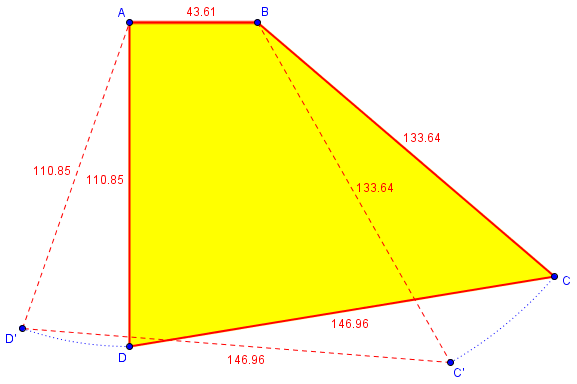Any one additional piece of information, such as an angle, would be almost enough to fix the shape. But only if we know it’s convex. Here is another version, in green, that is concave but has the same sides and one angle (clearly with a smaller area, namely 6140.14):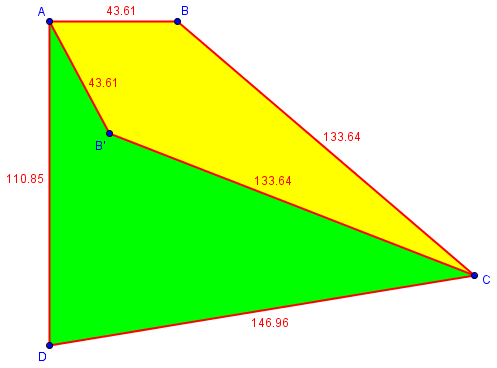So, in general, we will really need 6 facts to determine a quadrilateral.

## Looking at the extremes

For another illustration of this idea, consider this question from 2008:

Do Figures with Equal Sides Have the Same Area?

If you have a rectangle (figure A), with sides X and Y and Area = X x Y, and you do not change the length of the sides but change the angle formed by sides X and Y (i.e. decrease from 90 to 85 degrees)to make figure B, why is the area of figure B (calculated by the formula 1/2 B x H) now less than figure A?  This seems counter intuitive as the length of the sides of both figures A and B are still identical.

I replied to this more general question, with a similar example in a simpler case:

It's only counter-intuitive if your "intuition" wrongly assumes that figures with the same sides should have the same area.  There are several ways to train your intuition to see that the true result is perfectly natural.

First, imagine a rectangle made of jointed pieces of metal, hinged at the corners.  It starts out as a rectangle,

o------------------o
|                  |
|                  |
|                  |
|                  |
o------------------o

and then becomes

o------------------o
/                  /
/                  /
/                  /
o------------------o

which is not quite as high but may look to you like about the same area.  Push it over farther and keep watching:

o------------------o
/                  /
/                  /
o------------------o

o------------------o
/                  /
o------------------o

o------------------o
o------------------o

o------------o-----o------------o

Now its area is zero!  Is there any doubt that the area has been changing all along?  It just wasn't so obvious when you didn't push it so far.

This is a technique used by mathematicians: to check whether something is likely to be true in all circumstances (e.g. the idea that the area should not change), push it to the extreme and see if it still makes sense.

Another way to make this a little more intuitive is an approach that leads to calculus.  Think of a rectangle as a side view of a stack of cards:

-----------------------
-----------------------
-----------------------
-----------------------
-----------------------
-----------------------

If you push this over so that the side slants, it keeps the same height, rather than losing height as our other rectangle did; since it is still made of the same cards, this new figure must have the same area:

-----------------------
-----------------------
-----------------------
-----------------------
-----------------------
-----------------------

This time, no matter how far you push it, the height remains the same (though the pile would get a little unstable), and the area remains the same.

-----------------------
-----------------------
-----------------------
-----------------------
-----------------------
-----------------------

Moreover, the length of the slanting side increases; so you can see that if you push a rectangle over and keep the length of that side the same, you'll be losing area--some cards have to be taken out in order to preserve the length.

Does that help?

We discussed training your intuition in the post When Math Doesn’t Make Sense.

Now let’s find some actual areas, where we do have enough information!

## Four sides are enough for a trapezoid

Here is a nice question from 2008 that will make use of our ideas from last time:

Area of Trapezoid Given Only the Side Lengths

How would you find the area of a trapezoid without the height, but when all sides are given and they don't tell you which sides are parallel?

Ex. given trapezoid has side lengths of 1,2,3, and 4.  Find the area.

How would you find the area without the height?

We know that we can find the area of a trapezoid using the lengths of the two bases and the height (even though that is not enough to determine its shape!), using the formula $$K = \displaystyle \frac{h(b_1 + b_2)}{2}$$.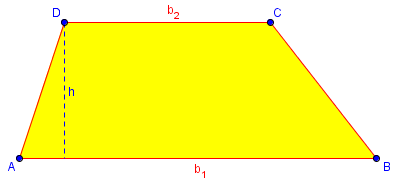So the four sides ought to be enough … except for that part about not knowing which sides are parallel. Hmm …

Interesting question!

You can find the area without knowing the height ahead of time, but you have to know which sides are parallel.  Different pairs of parallel sides may yield different areas.

To find the area given only the sides AND which are parallel, you can draw a parallelogram within the trapezoid:

+---------+
/         /  \
/         /     \
/         /        \
+---------+-----------+

You will now know all three sides of the triangle, from which you can find its height.

That is, we could use Heron’s formula to find the area of the triangle, and then use the triangle’s height to find the area of the parallelogram (or just plug that height into the trapezoid formula). We’ll be carrying that out once we figure out the sides below. I started exploring, by making a hopeful guess:

Let's try doing that with the obvious first choice of 2 and 4 for the parallel sides:

2
+---------+
/         /  \
1/        1/     \3
/   2     /   2    \
+---------+-----------+
4

Looking closely at the triangle, we see that this is impossible!  The Triangle Inequality Theorem says that the sum of any two sides must be greater than the other side, but 1+2 = 3.  So the 2 and 4 sides can't be parallel.

Note how I drew a generic picture without regard to actual lengths, just to let me think about the process; but the picture as labeled is nonsense, as the triangle would really be a line segment. (Try drawing the figure to scale, if you don’t see what’s wrong.)

Next, I did some work to determine which configurations would yield a valid figure, which I’ll let you read on your own if you’re interested. Here is the result:

So there's only one way to place the sides that will work.  (That may not always be true!)

1
+----+
/    /  \
2/   2/     \3
/ 1  /   3    \
+----+-----------+
4

As I said, for a different set of numbers, it is conceivable that there could be more than one valid arrangement; but this problem worked out nicely.

Let’s find the area:

Now we need to find the height:

+
/: \
2/ :h  \3
/  :     \
+---+-------+
3

We can use the Pythagorean theorem to find the two parts of the base in terms of h, and then solve for h:

sqrt(2^2 - h^2) + sqrt(3^2 - h^2) = 3

Try solving this; you'll get a fairly nice radical expression after some pretty hard work.  Then you can use this in the formula for the area of a trapezoid to find the area you want.

An alternative way to get the area is to find the area of the triangle using Heron's formula (see our FAQ on Formulas) and then add on the area of the parallelogram.

You may recognize that in the last post on Heron’s formula, the method I used here to find the height was the most difficult of three I demonstrated. Here is the work:

$$\sqrt{4 – h^2} + \sqrt{9 – h^2} = 3\\ \sqrt{4 – h^2} = 3 – \sqrt{9 – h^2}\\ 4 – h^2 = 9 – 6\sqrt{9 – h^2} + 9 – h^2\\ 6\sqrt{9 – h^2} = 14\\ 9 – h^2 = \frac{49}{9}\\ h = \sqrt{\frac{32}{9}} = \frac{4}{3}\sqrt{2}.$$

Finally, the area:

$$K = \frac{h(b_1 + b_2)}{2} = \frac{1}{2}\frac{4}{3}\sqrt{2}(1 + 4) = \frac{10}{3}\sqrt{2}.$$

Call the method above the altitude-first method, or the radical equation method.

Here is my alternative, which we can call the area-first method, or the Heron method:

The triangle has sides 2, 3, and 3, so $$\displaystyle s = \frac{2+3+3}{2} = 4$$, and by Heron’s formula its area is $$\sqrt{4(4-2)(4-3)(4-3)} = \sqrt{4\cdot2\cdot1\cdot1} = \sqrt{8} = 2\sqrt{2}.$$

Since this is equal to $$\frac{1}{2}bh = \frac{3}{2}h,$$ we have $$h = \frac{2}{3}A = \frac{2}{3}2\sqrt{2} = \frac{4}{3}\sqrt{2}$$ as before, and we get the same area.

## Alternatives for the trapezoid

Five years later, we got this comment from a reader, Denis:

There is an easier way to finish solving the problem than either way you suggested:

1
+----+
/|    | \
2/ |    |h  \3
/  |    |     \
+---+----+-------+
3-x   1     x
. . . . 4 . . . .

Using the left triangle:  h^2 = 2^2 - (3 - x)^2
Using the right triangle: h^2 = 3^2 - x^2

Combine these equations, you can solve for x, then find h and the area.

This is similar to a method from last time in which I deferred finding the altitude of the triangle, leaving me with a mere polynomial to solve. Call this the base-first method, or the polynomial method.

Here is the work:

$$4 – (3 – x)^2 = 9 – x^2\\ -5 + 6x – x^2 = 9 – x^2\\ 6x = 14\\ x = \frac{7}{3}.$$

Then we find h:

$$h = \sqrt{3^2 – x^2} = \sqrt{9 – \frac{49}{9}} = \sqrt{32}{9} = \frac{4}{3}\sqrt{2}.$$

Again, we got the same area.

This triggered further thoughts on my part:

Yes, that is much easier than my radical equation. It's a good example of coming at a problem from a different direction -- in this case, starting with two variables rather than one so that you have more freedom in how to solve it, then seeing that eliminating h (resulting in a polynomial) is easier than eliminating x (resulting in radicals). My equation actually amounts to doing the latter, because I essentially turned your equations into

3 - x = sqrt(2^2 - h^2)
x = sqrt(3^2 - h^2)

Then I added them together. (My picture contains the same two triangles as yours.)

But there are more ways to do it:

I also notice that my alternative idea can be improved, for this particular case, because my triangle happens to be isosceles:

+
1/  \
+ .  \3
1/    h  .\
+-----------+
3

We can first find the area of the triangle, by taking base 2 and finding the height to that base, as shown above, by the Pythagorean theorem.

+
/: \
2/ :h  \3
/  :     \
+---+-------+
3

Then, taking 3 as the base as shown here, we can use the known area to find the height h, and use that to find the area of the parallelogram.

Carrying this out, the first “height” is $$\sqrt{3^2 – 1^2} = \sqrt{8} = 2\sqrt{2},$$ so the area of the triangle is $$K = \frac{1}{2}bh = \frac{1}{2}2\cdot2\sqrt{2} = 2\sqrt{2}.$$ The rest of the work follows as in the Heron method. Call this the isosceles special case method.

Can you find more ways to do it?

## The biggest quadrilateral with given sides

There is one more thing to be said about trying to find the area of a quadrilateral given only the sides: We can at least determine the largest possible area. I’ve occasionally done that for someone who only knew the sides of their property; often the area will be reasonably close to the maximum, so that’s worth telling them. Here is one of two discussions we have had on this, from 2001:

Maximum Quadrilateral Area

Here is the question: Given a quadrilateral with sides of lengths a, b, c and d, prove that its area is maximized when opposite angles are supplementary.

I have sat on this question for weeks. I tried calculus, using area as a*b*sin(angle between) and taking the derivative. I got stuck with too many variables. I have often seen this fact, but not how to prove it.

My last resort? Dr Math!

Here is a picture: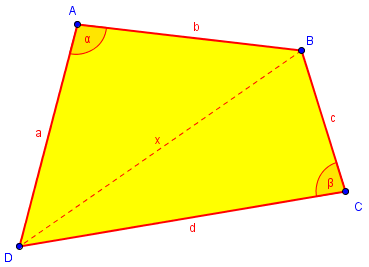Doctor Rick took up the challenge:

It can be proved using calculus. As you suggest, you start with the area formula:

K = (1/2)a*b*sin(alpha) + (1/2)c*d*sin(beta)

where alpha is the angle between sides a and b, and beta is the angle between sides c and d. The problem of too many variables is solved by using the Law of Cosines to relate alpha and beta. The diagonal x joining the other two vertices satisfies

x^2 = a^2 + b^2 - 2*a*b*cos(alpha)

from the one triangle, and

x^2 = c^2 + d^2 - 2*c*d*cos(beta)

from the other. Equating the two right sides, we get

cos(beta) = (c^2 + d^2 - a^2 - b^2 + 2*a*b*cos(alpha))/(2*c*d)

Now we have beta in terms of alpha, and might have plugged that directly into the formula for area, but that would be monstrous. Instead, we can keep it separate:

Take the derivative of cos(beta) with respect to alpha. Then go back to the area formula, and express sin(beta) as

sin(beta) = sqrt(1 - cos^2(beta))

Take the derivative of K with respect to alpha, using the formula for the derivative of cos(beta) with respect to alpha where you need it. You'll end up with

dK/d(alpha) = a*b/(2*sin(beta))*sin(alpha+beta)

All of this was given as instructions to Martin so he could do the work himself, but it’s long past the statute of limitations, so let’s do it for him.

First, the derivative of $$\cos\beta$$: $$\frac{\mathrm{d} }{\mathrm{d} \alpha}\cos\beta = \frac{\mathrm{d} }{\mathrm{d} \alpha}\frac{c^2 + d^2 – a^2 – b^2 + 2ab\cos\alpha}{2cd} = -\frac{ab}{cd}\sin\alpha$$

Now, we write the area as $$K = \frac{1}{2}ab\sin\alpha + \frac{1}{2}cd\sqrt{1 – \cos^2\beta}$$, so its derivative is $$\frac{\mathrm{d}K }{\mathrm{d} \alpha} = \frac{1}{2}ab\cos\alpha + \frac{1}{2}cd\frac{-2\cos\beta}{2\sqrt{1 – \cos^2\beta}}\cdot -\frac{ab}{cd}\sin\alpha =\\ \frac{1}{2}ab\left(\cos\alpha + \frac{\cos\beta}{\sin\beta}\sin\alpha\right) = \frac{1}{2}ab \frac{\sin(\alpha+\beta)}{\sin\beta}.$$

The derivative is zero when alpha+beta = 180 degrees. With a bit more work, I'm sure you can show that this condition gives the maximum of K.

So we have the condition for a possible maximum area, which turns out to be, in fact, the maximum.

Doctor Rick ended by referring to the following page from 2000, which gives a geometric/trigonometric approach to the question of maximum area:

Bretschneider's Theorem and Cyclic Quadrilaterals

My Calculus III professor brought up this problem in class: Prove that maximizing any quadrilateral with sides ABCD means inscribing it into a circle.

I used Bretschneider's theorem, which states that if s = (a+b+c+d)/2 and A and C are opposing angles in the quadrilateral, then the area of the quadrilateral is

sqrt((s-a)(s-b)(s-c)(s-d)-((cos((A+C)/2)^2)).

When this quadrilateral was inscribed, I saw that the opposing angles would be supplementary, and therefore the area would be maximized because the cosine term would be 0.

My professor patted me on the back and said, "Now prove the theorem. And while you're at it, explain why defining a quadrilateral with supplementary opposing angles means that it is inscribable into a circle."

Any insight on proving this theorem would be helpful. I do not know where to start. Also I know that a quadrilateral inscribed in a circle has opposing angles that add to 180 degrees, but I don't know how to show the converse, that if a quadrilateral has supplementary opposing angles, then it can be placed inside a circle.

As Rachel said, Bretschneider’s formula, for the area of any quadrilateral given four sides and two opposite angles (note: 6 facts again), is $$K = \sqrt{(s-a)(s-b)(s-c)(s-d)-\cos^2\frac{A+C}{2}};$$ since the squared cosine is always positive, this area is smallest when the cosine is zero, so that $$\frac{A+C}{2}$$ is 90°, so that $$A+C = 180°$$.

I will omit Doctor Rob’s proof of the theorem (this post is already long enough), but here is what he said about the quadrilateral being cyclic (inscribed in a circle):

To show that when opposite angles are supplementary the quadrilateral is cyclic is not too hard. Pick one of the two angles, say <ABC, and draw the diagonal AC. This forms a triangle ABC. Draw the circumcircle of this triangle. Then the arc AC has measure double that of of <ABC.

Now consider line CD. This intersects the circle at point E. Draw EA. Then <CEA has measure half that of arc CA. Since arcs CA and AC have total measure 2 pi rad, or 360 degrees, <CEA and <ABC have total measure pi rad, or 180 degrees.

Now since <ABC and <CDA are supplementary, and <ABC and <CEA are also supplementary, then <CEA = <CDA. But since the angles of triangle CDE add up to pi rad or 180 degrees, this implies that points E and D coincide, and so D lies on the circle. (This uses the fact that any exterior angle of a triangle is the sum of the two other interior angles.)

So the largest quadrilateral for a given set of sides has all its vertices on a circle.

Let’s apply this to the first problem we looked at. I drew two possible convex configurations of the property, whose areas (according to GeoGebra, which I used to draw it) are 9935.25 (solid lines) and 10,405.58 (broken lines) respectively. Here is the largest possible area, 10,480.49: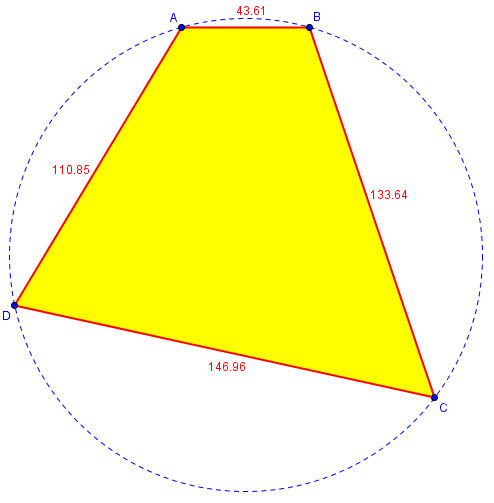We can use Bretschneider’s formula with the square term removed, which is called Brahmagupta’s formula, to verify the area: $$s = \frac{1}{2}(43.61+133.64+146.96+110.85) = 217.53\\ K = \sqrt{(s-a)(s-b)(s-c)(s-d)} = \sqrt{(173.92)(83.89)(70.57)(106.68)} = 10,480.49.$$

By the way, did you notice how similar Brahmagupta’s formula is to Heron’s formula? We get the latter if we just shrink the fourth side of the quadrilateral to 0. That makes sense, doesn’t it? And this formula is even more memorable than Heron’s.

It turns out also that the fact we are discussing extends to any polygon: the greatest area for a given set of sides is that of the cyclic polygon. Unfortunately, I don’t know of a formula that will give that area directly.

Next time we’ll look into formulas based on coordinates of vertices; then we’ll have all the tools we need to work on finding the area of your yard.

This site uses Akismet to reduce spam. Learn how your comment data is processed.